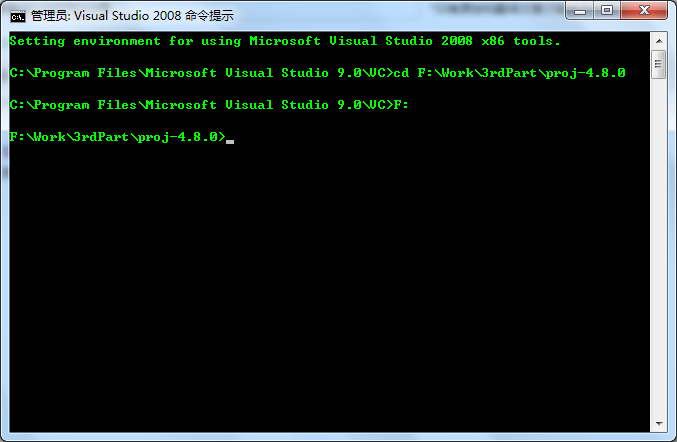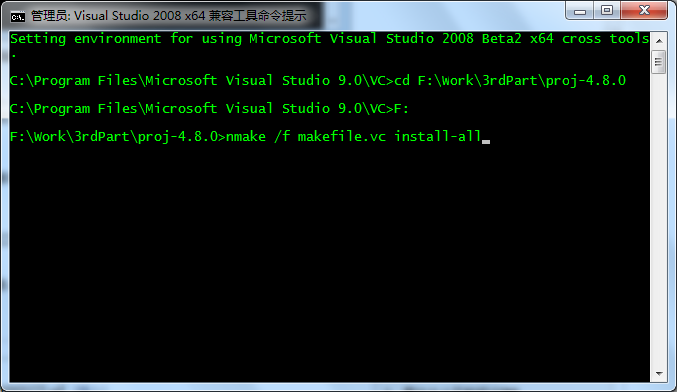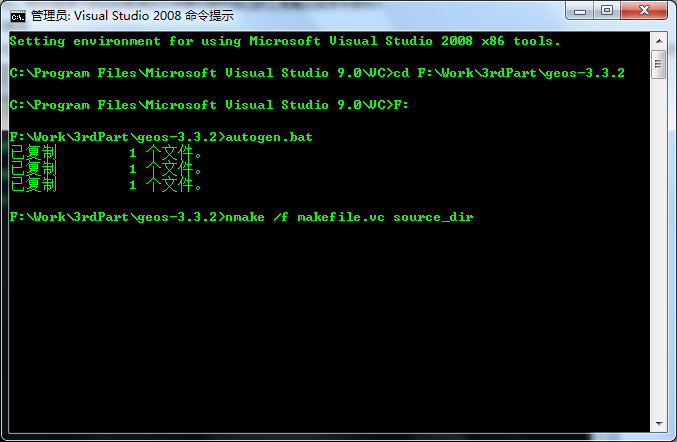# proj4.8编译

PROJ4的最新版本是4.8，官网地址为：http://trac.osgeo.org/proj/。从官网下载PROJ4的源代码，解压到文件夹中，如F:\Work\3rdPart\proj-4.8.0。

1、正常编译RELEASE版本nmake /f makefile.vc install-all

nmake /f makefile.vc clean

nmake /f makefile.vc install-all DEBUG=11、正常编译RELEASE X86版本atuogen.bat

nmake /f makefile.vc src_dir

[cpp] view plaincopyprint?
<span style=”color: rgb(0, 0, 153);”>/*private*/
double
BufferOp::precisionScaleFactor(const Geometry *g,
double distance,
int maxPrecisionDigits)

const Envelope *env=g->getEnvelopeInternal();
double envMax = std::max(
std::max(fabs(env->getMaxX()), fabs(env->getMinX())),
std::max(fabs(env->getMaxY()), fabs(env->getMinY()))
);
double expandByDistance = distance > 0.0 ? distance : 0.0;
double bufEnvMax = envMax + 2 * expandByDistance;
// the smallest power of 10 greater than the buffer envelope
int bufEnvPrecisionDigits = (int) (std::log(bufEnvMax) / std::log(10.0) + 1.0);
int minUnitLog10 = maxPrecisionDigits – bufEnvPrecisionDigits;
double scaleFactor = std::pow(10.0, minUnitLog10);
return scaleFactor;
}</span>

```/*private*/
double
BufferOp::precisionScaleFactor(const Geometry *g,
double distance,
int maxPrecisionDigits)
{
const Envelope *env=g->getEnvelopeInternal();
double envMax = std::max(
std::max(fabs(env->getMaxX()), fabs(env->getMinX())),
std::max(fabs(env->getMaxY()), fabs(env->getMinY()))
);

double expandByDistance = distance > 0.0 ? distance : 0.0;
double bufEnvMax = envMax + 2 * expandByDistance;

// the smallest power of 10 greater than the buffer envelope
int bufEnvPrecisionDigits = (int) (std::log(bufEnvMax) / std::log(10.0) + 1.0);
int minUnitLog10 = maxPrecisionDigits - bufEnvPrecisionDigits;

double scaleFactor = std::pow(10.0, minUnitLog10);

return scaleFactor;
}```

2、编译DEBUG版本

atuogen.bat

nmake /f makefile.vc clean

nmake /f makefile.vc src_dir DEBUG=1

3、编译X64版本